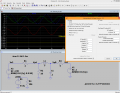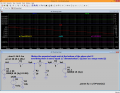# AC circuit: Find the current through the inductor

#### michgkou

Joined Oct 3, 2019
43

#### ci139

Joined Jul 11, 2016
1,873
you use DC stationary voltage sources -- Right-Click your voltage source and select Sine and set parameters
basically you have same time parameter for Sine Cosine so you need to sift the phase of one of these by 90° the cos is 90 deg earlier e.g. when sine is 0 for φ=2πft=2πt/τ then cos(φ) = sin(φ+90°) = sin(φ)·cos(π/2) + sin(π/2)·cos(φ) !!! but you need to check which way the phase is implemented in Spice --e.g.-- that the programmer hasn't used the "complementing logic" which would reverse the sign . . . if i remember right then sine with -90 deg shift in LTSpice satrts from -1 @ t=0 so the logic is "not complemented" and the formula in RED , above applies . . .

. . . also when using .BV (behavioural voltage source) or .funct directive the time is entered by parameter name time (not t as in your case) also 2t should be written as 2*time

+ if you use .V source the frequeny is derived from 2t=2πft → → f = (2t)/(2πt) = 1/π ≈ 381.31mHz ← you enter this in Spice as 381m31 or .38131 or {1/(4*atan(1))}
/// the last is because in places the LTSpice interprets (it's built in constant) pi as π but another context it interprets it as 180(°) !!! -- but 4*atan(1) always evaluates as π -- so you don't need to test it out /!\ but this is again when the Spice doesnot expect frequency in degrees as on bode plot display formula . . .
_Last edited:
•michgkou

#### MrAl

Joined Jun 17, 2014
9,781
What are you analyzing for?
Is it the voltage at the two nodes or some current(s) ?

#### MrAl

Joined Jun 17, 2014
9,781
When you use a circuit simulator for purely AC quantities you have to be aware of a few things.
1. The AC quantity does not appear as purely AC until after all the exponentials have died out.
2. The phase shifts are relative to t=0 which means you have to use a reference phase to be able to measure the phase shift after all the exponentials have died out.

#1 simply means that you have to wait some time in order to read the peak value of the output sinusoid(s).
#2 simply means that you should plot a reference phase like 20*sin(w*t) so you can measure the phase shift after the exponentials have all died out.

The key point is to determine when all the exponentials have died out. This usually is not too hard to do but it could be some long time so you have to be aware that it is easy to make a mistake thinking they all died out when they havent.
To figure this out, look for wild wave behavior followed by what looks like a pure sinusoidal wave centered at zero voltage. If the upper most peak is farther above zero than the lower most peak then the exponential is still in effect so you will have to wait longer. This means extending the analysis time.

Just to help a little here, 20 seconds for this problem should be enough time. Then you can read the phase shift (using the reference phase mentioned above) and the peak amplitude so you can compare with your numerical calculation results. They should match quite closely.

•michgkou